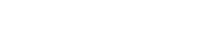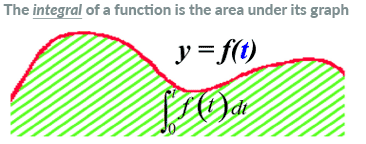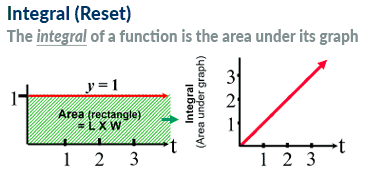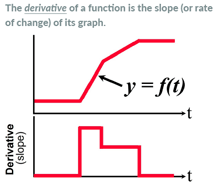# Fundamentals of PID Control

• By Jon Monsen, PhD, PE
• June 26, 2023
• Features

## Proportional-integral-derivative (PID) is the most common industrial technology for closed-loop control.

A proportional-integral-derivative (PID) controller can be used to control temperature, pressure, flow, and other process variables. A PID controller combines proportional control with additional integral and derivative adjustments to help a controller automatically compensate for system changes.

The basic control mode is “proportional,” which “uses error to reduce error.” The diagram in Figure 1 illustrates how proportional functions. Shown is a mechanical proportional controller consisting of a float that operates a valve to maintain the level at the desired setpoint (SP) of 50 percent.

Consider what would happen if the fulcrum point was set at the left most position (for now ignore the other two fulcrums). The operation of the controller is graphically represented by the blue line with the steepest slope. The horizontal axis is the percent error from the setpoint of 50 percent full. The vertical axis is valve position. If this were a pneumatic or electronic controller, the vertical axis would be the controller output signal, but because this is a mechanical controller, the controller output is the valve position.

The definition of gain of any device is “change in output divided by the corresponding change in input.” For a proportional controller, the output is the controller output (abbreviated “C.O.”). The input is the error between setpoint and measurement. Throughout this article, “e” is the error between the setpoint and the process variable measurement. The symbol for gain is usually “K.” Here, the focus is on “proportional” gain, so the symbol is “K” with a subscript “p” for “proportional.”

For the fulcrum in the left-most position and the resulting graph of error versus valve position with the steepest slope when the error changes from minus 25 percent to plus 25 percent (a total of 50 percent), the valve position changes by 100 percent. The proportional gain is 100 divided by 50, or 2. With the fulcrum in this far left position, the result is the largest change in valve position for a given change in float position. Of the three fulcrum positions, this one will give the highest gain (or the greatest sensitivity).

If the fulcrum is moved to the center position, the valve travel does not change as much for the same amount of error, and the action of the controller is represented by the red line on the graph. In this case, a change in error from minus 50 percent to plus 50 percent (a total of 100 percent) causes the valve travel to change by 100 percent. So, the gain is now 100 divided by 100, or 1.

Moving the fulcrum to the far-right position yields the least sensitivity. The controller’s action is represented by the graph with the green line. In this case, a change in error from minus 50 percent to plus 50 percent (a total of 100 percent) causes the valve travel to change by 50 percent. The gain is now 50 divided by 100 or 0.5.

Sometimes, instead of talking about proportional gain, people talk about “proportional band,” abbreviated “P.B.” in the figure. Mathematically, the proportional band is the reciprocal of the proportional gain times 100 and expressed as a percent.

“Offset” is the difference between the setpoint and the actual measurement—in this case, tank level. If the valve is not in the right position for the load from the very beginning, or once there is a change in load (in this example, the flow out of the tank), there will be some offset. For the valve to open farther so that the inflow will match the new higher outflow, the float will have to be lower than it was originally.

This is a characteristic of all controllers that only have the proportional mode. The proportional mode uses the error to reduce the error, so it is necessary for there to be an error (in control terms called “offset”) for the error reduction to occur.

The two graphs on the left in Figure 2 show the relationship between the measurement and the controller output from a proportional controller. As soon as an error (e) occurs between the measurement and the setpoint, the controller output changes to exactly mirror the error, except that the magnitude of the controller output change depends on the proportional gain of the controller. In this case, the proportional gain is less than one since the change in output is less than the change in error. The direction of the controller output change is chosen to be in the direction that will tend to correct the error. The graphs on the left show the “open loop” interaction between error and controller output—in other words, how the controller responds to an error—but the output is not connected to the process. Shortly, graphs will show what happens when the loop is closed, and the controller is regulating the process.

The graph on the right of Figure 2 shows how a first-order process would respond to a step change in load while being controlled by a proportional controller. The important point here is that with proportional control, the error is being used to reduce the error, so there will always be some residual error, which we call offset.

The water heater shown in Figure 3 illustrates the behavior of the various control modes. Although the water heater consists of several dynamic subsystems (control valve, the heating vessel, the temperature element, and the temperature transmitter), when a step test is performed with the controller in manual, the response (for all practical purposes) can be treated as a first-order response with dead time.

To get a reference point for evaluating the performance of the controller, the controller has been left in manual, and then a step change in load was introduced. This was done by suddenly decreasing the demand for hot water. Since the steam flow does not change, the measured temperature increases to a new value following the approximately first-order plus dead time response is shown by the green line in Figure 4.

The controller is next placed into automatic mode with a small amount of proportional gain (Kp = 0.3). The controller reduces the error slightly, but there remains a large residual error, or offset.

Increasing the proportional gain to 1.5 causes a smaller offset. Further increasing the proportional gain to 3 gives an even smaller error and thus better control. Note that there is a small oscillatory transient at first. At this point, it is tempting to assume that the higher the gain, the better the control, and that it might be possible to decrease the offset to a very small value by setting a very large proportional gain. However, when we try increasing the gain to see what happens, at some point, with increasing proportional gain, the system becomes unstable.

## Integral, when proportional gain is not enough

If some offset cannot be tolerated, some way of supplementing the proportional control mode must be ascertained. To remove the offset of the proportional control mode, the integral (sometimes called reset) mode is introduced.Figure 5. Definition of the mathematical integral. In calculus, the “integral” of a function is “the area under the graph” of that function. Figure 5 shows an arbitrary complex function of time and its graph. If the exact function that produces this graph is known, the area under the curve could be determined, but it often takes methods that students spend a whole year in calculus learning. Fortunately, this is a simple function, and one that is easy to calculate the area under the graph without any advanced techniques is all that’s needed to make sense out of how the integral control mode works.Figure 6. In an open loop, the integral of the error continues to increase with time. A time function whose value is always 1.0 is shown in Figure 6. Since the function’s value remains constant, the area under its graph is always a rectangle, and the area of a rectangle is easy to calculate without using advanced techniques.

Imagine starting at time equal to zero, and then watching what happens as time progresses. At exactly time = zero, the length of the rectangle is zero and its width is 1. The area is zero times one or zero. After one second has passed, (time is now equal to 1 second), the length of the rectangle is 1, and the width is 1, so the area is 1 times 1, or 1. The graph on the right shows how the integral (area under the curve in the left-hand graph) has changed during the first second. As time continues to progress and the area of the rectangle increases, the graph on the right continues to track what the rectangle’s area is at any moment. Since the area under the curve is increasing in a linear fashion with time, the graph of the integral is a ramp, also increasing in a linear manner with time. Adding the integral control mode to the proportional mode makes it possible to remove the offset left by the proportional mode. This controller has been configured so that both the proportional and integral actions are downward instead of upward because that is the direction that will eliminate the error.

Figure 7 shows how a proportional plus integral controller reacts to a step change in load in an open loop (the controller output is not connected to the process). At the moment the error first occurs, there is an immediate proportional action in the controller output. Then the controller output starts ramping down (integral action) in proportion to the area under the graph (error times the constantly increasing time). The parameter that is set into the controller to tell it how strongly the integral action is to act on the controller output is called the “integral time,” or TI. The integral time is the time it takes the integral action to repeat the correction produced by the proportional action. A short integral time means the controller ramps its output quickly to eliminate the error, and a long integral time means the output ramps slowly to eliminate the error (or offset). The units are minutes (or seconds depending on the controller manufacturer) per repeat. Some controller manufacturers use “integral gain,” which is the reciprocal of integral time. In that case, the units are repeats per minute (or second).

Figure 8 is the same graph as Figure 4, but starting when the proportional controller was running in closed loop with a proportional gain of 1.5. When considering the effect of various values of proportional gain, there was better (but slightly oscillatory) control with a gain of 3, but because it is known that this integral action is destabilizing, and would have resulted in an oscillatory response, the slightly lower proportional gain was chosen for this example.

In Figure 8, some integral action has been added. Initially, the proportional action eliminates part of the error, then the integral, or reset, action continues to drive the control valve until all the offset has been removed. In closed loop, once all the error has been eliminated, the proportional action settles out at the new value required to hold the error at zero, and since there is no error, the integral of the error is zero, thus there is no further integral action.

## Derivative, when error must be eliminated faster

The next question might be: can the integral time be decreased to make the error be eliminated more quickly? As with proportional gain, some integral is good, but too fast an action destabilizes the process.

Before discussing the derivative (sometimes called rate) control mode, consider this brief review of the meaning of the derivative. In calculus, the derivative of a function can be interpreted as the instantaneous slope of that function’s graph at any point. Students spend the better part of a year in calculus class learning how to do this for all sorts of functions. Fortunately, for purposes of discussing the derivative control mode, all that’s needed is to review the behavior of the derivative of straight lines.Figure 9. Definition of the mathematical derivative. The graph of a function of time whose shape consists entirely of straight lines with different slopes is shown in Figure 9. Starting at time = zero and continuing for a while, the functions value is zero. Its slope is also zero and thus its derivative is zero, as shown in the lower graph. The value of the function suddenly begins increasing at a steady rate. Its derivative (slope) instantly becomes a finite (and constant) value, again portrayed in the lower graph. Next, the function continues to increase, but at a lesser rate (its slope still has a finite and constant value, but a smaller one). Again, this smaller, but constant rate of change (slope or derivative) is graphed in the lower graph. Finally, the time function stops growing, and levels off at a constant value. At this point, there is no more change in the function’s value (its rate of change or slope or derivative becomes zero) and is graphed on the lower graph of derivative as a derivative of zero.

When examining the proportional control mode and the integral control mode, their actions are based on the assumption that a fairly fast control process was discussed. The discussion was made simpler (without loss of meaning) by assuming that upon a process disturbance, the measurement made a step increase (like in the line in the upper graph in Figure 2).

Some processes, such as the water heater used as an example, respond slowly to process upsets. In such a case, the ramp in the upper graph of Figure 10 is a simplified but more realistic depiction of what happens when in open loop, that is, the controller output is not connected to the process. In this example, the process upset could have been a nearly instantaneous decrease in the demand for hot water from the water heater. At the point where the ramp just starts, the damage has already been done and the process is heading toward a large error. The problem here is that because the process responds slowly, the controller does not immediately see the large error that is on its way. The controller only sees a small error at first.

In the upper graph of Figure 10, the error starts out being very small, and with proportional only control, the controller’s output would only be a small correction at first represented by the sloping dashed line. In a slow process, the disturbance was likely a large one, but because the process responds slowly, the large disturbance is not seen right away. At the point where the measurement begins to deviate from the setpoint, the slope of the measurement (its derivative) makes a sudden jump from zero to a value equal to the slope of the measurement’s graph. This provides an instantaneous jump in the controller output, in anticipation of the large error that isn’t seen yet but is coming. The proportional correction gets added to the derivative correction, so that after the initial “boost” of the derivative, the controller output continues with a correction proportional to the error. (To avoid unnecessary complication to the explanation, the integral action was not included in the discussion of derivative action.)

The parameter set into the controller to tell it how strongly the derivative action is to act on the controller output is called the “derivative time,” or TD. The derivative time is the time it would have taken the proportional action to produce the correction that was immediately produced by the derivative action. (This description presumes the error remains constant, independent of any control action.) A short derivative time means the controller adds only a small derivative output to anticipate a future error. A long derivative time means the controller adds a large derivative output to anticipate a future error. The units are minutes (or seconds depending on the controller manufacturer). Also, some controller manufacturers use “derivative gain,” which is the reciprocal of derivative time. In that case, the units are 1 divided by minutes (or seconds).

Some controllers take the derivative from the measurement rather than the error. This prevents a large derivative correction (called a “derivative kick”) if the setpoint is manually changed suddenly. Noise spikes in a noisy measurement can cause undesired large outputs form the derivative mode. Derivative correction must be used with caution when the measurement is noisy. Filtering the signal before it goes to the derivative function can help.

In Figure 11, the upper two traces show what could be accomplished with proportional only and with proportional plus integral (P+I). Here, derivative (P(1.5) + I + D) has been added to the earlier P+I to further reduce the maximum error.

The derivative mode—unlike the integral mode, which tends to destabilize control—adds stability. Because of this, it is possible to increase the proportional gain from 1.5 to 2. If the gain had been increased to 2 with just integral, there would have been a response with too much oscillation in it. However, with the stabilizing effect of the derivative, the result is a response that is better than what we would have gotten with just P+I or with P+I+D using the proportional gain that would have been optimum had the derivative not been added.

Derivative controls may also be sensitive to fast, short-term process signals, including sensor noise or process noise. For example, if there were waves in the tank, the level signal would be constantly moving up and down, and the derivative action could amplify those waves into valve movements. For this and other reasons, derivative action is much less common in practice, and P+I controllers are most often seen.